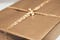# In Java, mind the difference between primitives and their wrappersPhoto by freestocks on Unsplash
`char[] letters = {'a', 'b', 'c', 'd'};int[] primes = {2, 3, 5, 7};String[] muskNames = {"Aramis", "Athos", "D'Artagnan", "Porthos"};Exception[] exceptions = {new IndexOutOfBoundsException(),             new InputMismatchException(),            new NullPointerException(),             new RuntimeException()};`
`ArrayList<String> muskNames = new ArrayList<>();muskNames.add("Aramis");muskNames.add("Athos");muskNames.add("Porthos");`
`ArrayList<Integer> primes = new ArrayList<>();primes.add(new Integer(2));primes.add(new Integer(3));primes.add(new Integer(5));primes.add(new Integer(7));`
`ArrayList<Integer> primes = new ArrayList<>();primes.add(2);primes.add(3);primes.add(5);primes.add(7);`
`package org.oeis.primes;import java.util.ArrayList;public class PrimeLister {        private static final ArrayList<Integer> PRIMES =                                                  new ArrayList<>();    private static int currThresh;        static {        PRIMES.add(2);        PRIMES.add(3);        PRIMES.add(5);        PRIMES.add(7);        currThresh = 10;    }        // STUB TO FAIL THE FIRST TEST    public static ArrayList<Integer> listPrimes(int threshold) {        ArrayList<Integer> selPrimes = new ArrayList<>();        return selPrimes;    }    }`
`package org.oeis.primes;import java.util.ArrayList;import java.util.Arrays;import org.junit.Test;import static org.junit.Assert.*;public class PrimeListerTest {        @Test    public void testListPrimes() {        System.out.println("listPrimes");        int threshold = 10;        Integer[] smallPrimes = {2, 3, 5, 7};        ArrayList<Integer> expResult =                        new ArrayList<>(Arrays.asList(smallPrimes));        ArrayList<Integer> result =                                  PrimeLister.listPrimes(threshold);        assertEquals(expResult, result);    }    }`
`    public static ArrayList<Integer> listPrimes(int threshold) {        ArrayList<Integer> selPrimes = new ArrayList<>(PRIMES);        return selPrimes;    }`
`    @Test    public void testListPrimesTo100() {        int threshold = 100;        Integer[] smallPrimes = {2, 3, 5, 7, 11, 13, 17, 19, 23, 29,                                 31, 37, 41, 43, 47, 53, 59, 61, 67,                                 71, 73, 79, 83, 89, 97};        ArrayList<Integer> expResult =                        new ArrayList<>(Arrays.asList(smallPrimes));        ArrayList<Integer> result =                                  PrimeLister.listPrimes(threshold);        assertEquals(expResult, result);    }`
`    public static ArrayList<Integer> listPrimes(int threshold) {        int thresh = Math.abs(threshold);        if (thresh > currThresh) {            for (int n = currThresh + 1; n <= thresh; n++) {                double root = Math.sqrt(n);                boolean noDivisorFound = true;                int index = 0;                int p;                do {                    p = PRIMES.get(index);                    noDivisorFound = (n % p != 0);                    index++;                } while (p <= root && noDivisorFound);                if (noDivisorFound) PRIMES.add(n);            }            currThresh = thresh;        }        return new ArrayList<>(PRIMES);    }`
`    @Test    public void testPrimeListerCanTrim() {        int threshold = 80;        ArrayList<Integer> result =                                  PrimeLister.listPrimes(threshold);        System.out.println("PrimeLister reports " + result.size()                 + " primes between 1 and " + threshold);        threshold = 20;        Integer[] smallPrimes = {2, 3, 5, 7, 11, 13, 17, 19};        ArrayList<Integer> expResult =                        new ArrayList<>(Arrays.asList(smallPrimes));        result = PrimeLister.listPrimes(threshold);        assertEquals(expResult, result);    }`
`        if (thresh < currThresh) {            int trimIndex = PRIMES.size();            int p;            do {                trimIndex--;                p = PRIMES.get(trimIndex);            } while (p > thresh);            return new ArrayList<>(PRIMES.subList(0, trimIndex));        }`
`        if (thresh < currThresh) {            int trimIndex = PRIMES.size();            int p;            do {                trimIndex--;                p = PRIMES.get(trimIndex);            } while (p > thresh);            return new ArrayList<>(PRIMES.subList(0,                                                    trimIndex + 1));        }`

is a composer and photographer from Detroit, Michigan. He has been working on a Java program to display certain mathematical diagrams.

## More from Alonso Del Arte

is a composer and photographer from Detroit, Michigan. He has been working on a Java program to display certain mathematical diagrams.